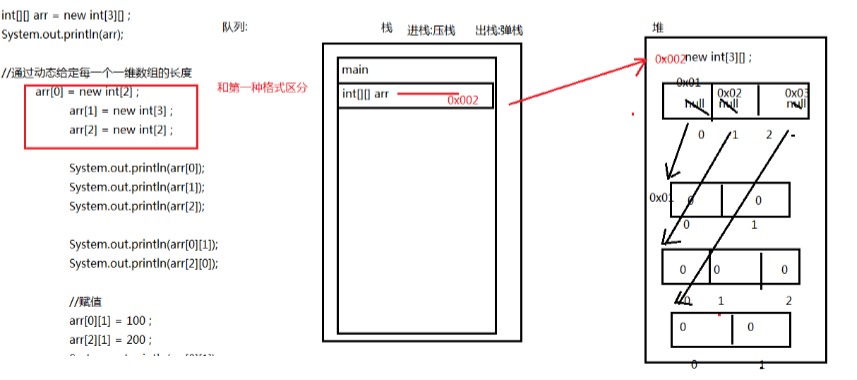## 二维数组的格式

### 五种定义格式：

int[][] arr = new int; //创建一个两行三列的数组 arr

int[] arr[] = new 数据类型 ;//创建一个两行三列二维数组arr

int arr[][] = new int[m][n] ;

int[][] arr =  new int[] ;
解释：格式4我们建立m个一维数组，每个一维数组的长度动态给定

动态给定一维数组长度的方法：
int = new int; //给定第1个一维数组的长度为2
int = new int; //给定第2个一维数组的长度为6
int = new int; //给定第3个一维数组的长度为3
这种格式我们无法省略m位的值，因为二维数组每个组成的一维数组都需要申请内存，所以必须得确定。
C语言与Java中二维数组最大的不同之处就是C语言是面向过程的思想，而Java是面向对象的思想，所以Java在定义二维数组时需要先申请一块内存。Java中二维数组的组成是 int--->int。即先在堆内存中开辟一个空间用来存放每个一维数组的地址值，再在堆内存中申请每个一维数组的堆内存。

## 二维数组的遍历

我们先静态初始化一个二维数组

```		int[][] arr = {{1,2,3},{3,3,3},{2,2,2,2,2},{1}};
```

```		for(int x = 0 ; x < arr.length ; x++) {//一维数组个数遍历
for(int y = 0 ; y <arr[x].length ; y++) {//一维数组的元素数遍历
System.out.print(arr[x][y]);
if(y == arr[x].length-1) {//如果是这个一维数组的最后一个元素，换行
System.out.println();
}
}
}```

我们在遍历二维数组的列数时，对于列数我们可以用arr[x].length得出，这个是我在初学时没有太在意的东西。下面是创建二维数组的内存变化图：## 例子：

```/***
* 练习:
公司年销售额求和
某公司按照季度和月份统计的数据如下：单位(万元)
第一季度：22,66,44
第二季度：77,33,88
第三季度：25,45,65
第四季度：11,66,99
* @author malaganguo
*
*/
class Summoney{
public static void Sumfest(int arr[][]) {
int sumfest = 0 ;
for(int x = 0 ; x < arr.length ; x++) {
for(int y = 0 ;y < arr[x].length ;y++) {
sumfest += arr[x][y];
}
System.out.println("第" + (x+1) +"季度的总营业额为："+sumfest+"元");
sumfest = 0;
}
}
public static void Sum(int arr[][]) {
int sum = 0;
for(int x = 0 ;x < arr.length ; x++) {
for(int y = 0; y < arr[x].length ; y++) {
sum += arr[x][y];
}
}
System.out.println("总营业额为:"+sum + "元");
}
}
public class ArrayTest2 {

public static void main(String[] args) {
int[][] arr = {{22,66,44},{77,33,88},{25,45,65},{11,66,99}};
Summoney.Sumfest(arr);
Summoney.Sum(arr);
}

}
```